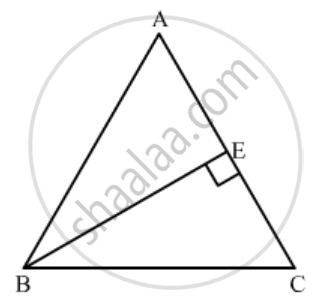Advertisement Remove all ads

# If E is a Point on Side Ca of an Equilateral Triangle Abc Such that Be ⊥ Ca, Then Ab2 + Bc2 + Ca2 = (A) 2 Be2 (B) 3 Be2 (C) 4 Be2 (D) 6 Be2 - Mathematics

MCQ

If E is a point on side CA of an equilateral triangle ABC such that BE ⊥ CA, then AB2 + BC2 + CA2 =

#### Options

• 2 BE2

• 3 BE2

• 4 BE2

• 6 BE2

Advertisement Remove all ads

#### Solution

In triangle ABC, E is a point on AC such that  BE ⊥ AC.

We need to find AB^2+BC^2+AC^2.Since BE ⊥ AC, CE = AE =

$\frac{AC}{2}$(In a equilateral triangle, the perpendicular from the vertex bisects the base.)

In triangle ABE, we have

AB^2 = BE^2+AE^2

Since AB = BC = AC

Therefore, AB^2=BC^2=AC^2=BE^2+AE^2

Since in triangle BE is an altitude, so  BE = (sqrt3)/2 AB

BE = (sqrt3)/2 AB

(sqrt3)/2 xxAC

(sqrt3)/2 xx 2AE= sqrt3AE

⇒ AB^2 + BC^2+AC^2= 3BE^2+3((BE)/sqrt3)^2

= 3BE^2+BE^2=4BE^2

Hence option (c) is correct.

Concept: Triangles Examples and Solutions
Is there an error in this question or solution?
Advertisement Remove all ads

#### APPEARS IN

RD Sharma Class 10 Maths
Chapter 7 Triangles
Q 47 | Page 136
Advertisement Remove all ads

#### Video TutorialsVIEW ALL 

Advertisement Remove all ads
Share
Notifications

View all notifications

Forgot password?
Course